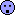## Indirect adjusted comparisons in BE [General Sta­tis­tics]

Hi Shuanghe, Mittyri, and nobody,

yep, you are all right. Nobody’s definition of “pragmatic” is correct. IMHO, here it should be called – following Wolfgang Pauli’s footprints – “not even wrong”.Pooling variances without weighting by the sample size is just false.

Quoting Shuanghe’s reference, Section Data analysis:

In line with recommendations by Gwaza et al., a pragmatic method based on t test was used […]. This method has been shown to give comparable results as the homoscedastic method.

Great. What Gwaza et al.* really wrote/recommended is this:

The [pragmatic] method based on a combination of both (SE calculation of the heteroscedastic method and degrees of freedom of the homocedastic method), which is the simplest numerical calculation, gives results similar to those obtained with the heteroscedastic approach, showing that the contribution of the different method of calculation of the degrees of freedom is negligible.
In conclusion, the homoscedastic method is recommended when performing adjusted indirect comparisons of bioequivalence studies—unless a clear difference in variances is observed in the data, which is unlikely.

Gwaza drives me nuts. What is “SE calculation of the heteroscedastic method”? There is only one correct way to pool variances. No, it’s not $$SE_d^2 = SE_1^2 + SE_2^2$$. The homo- and heteroscedastic methods differ only in the degrees of freedom.
• homoscedastic
$$\nu = n_1+n_2-2$$
• heteroscedastic
$$\nu \approx \frac{\left (\frac{s_{1}^{2}}{n_1}+\frac{s_{1}^{2}}{n_2}\right )^2}{\frac{s_{1}^{4}}{n_1(n_1-1)}+\frac{s_{1}^{4}}{n_2(n_2-1)}}$$
This is not the first paper written by regulators where the conclusions contradict the result.Comes handy cause most people don’t read beyond the abstract (men on a mission?). When I rank the width of the CIs given in the two tables:
1. The pragmatic method lies between the hetero- and homoscedastic methods.
2. The pragmatic method is much closer to the hetero- than to the homoscedastic method.
(a) and (b) agree what he wrote above. As a regulator why does he recommend the most liberal one (tightest CI)?
If I use the crappy formula I end up with CIs which are not slightly tighter than the ones of the hete­ro­scedastic method – as expected – but slightly wider. Puzzle not solved.
Since I’m dealing with HVD(P)s, where the CV jumps around like crazy between studies I prefer the heteroscedastic approach.

» OT again:Easy.

» Thanks a lot for implementing MathJax. Equations are looking much better. one puzzle though: command \LaTeX does do what it supposed to do.There is not \LaTex in MathJax. See there for examples (including a link to the MathJax site).

• Gwaza L, Gordon J, Welink J, Potthast H, Hansson H, Stahl M, García-Arieta A. Statistical approaches to indirectly compare bioequivalence between generics: a comparison of methodologies employing artemether / lume­fantrine 20/120 mg tablets as prequalified by WHO. Eur J Clin Pharmacol. 2012;68(12):1611–8. doi:10.1007/s00228-012-1396-1.

Dif-tor heh smusma 🖖
Helmut SchützThe quality of responses received is directly proportional to the quality of the question asked. 🚮
Science QuotesIng. Helmut Schütz